# RD Sharma Solutions for Class 10 Maths Chapter 4 Triangles Exercise 4.7

Baudhayan theorem also commonly known as the Pythagoras theorem is the key concept in this exercise. Students will get a clear picture of the concept when solving the problems of the exercise. To guide students in the right direction RD Sharma Solutions Class 10 is prepared by subject experts at BYJU’S. Additionally, the RD Sharma Solutions for Class 10 Maths Chapter 4 Triangles Exercise 4.7 PDF is provided below.

## RD Sharma Solutions for Class 10 Maths Chapter 4 Triangles Exercise 4.7 Download PDF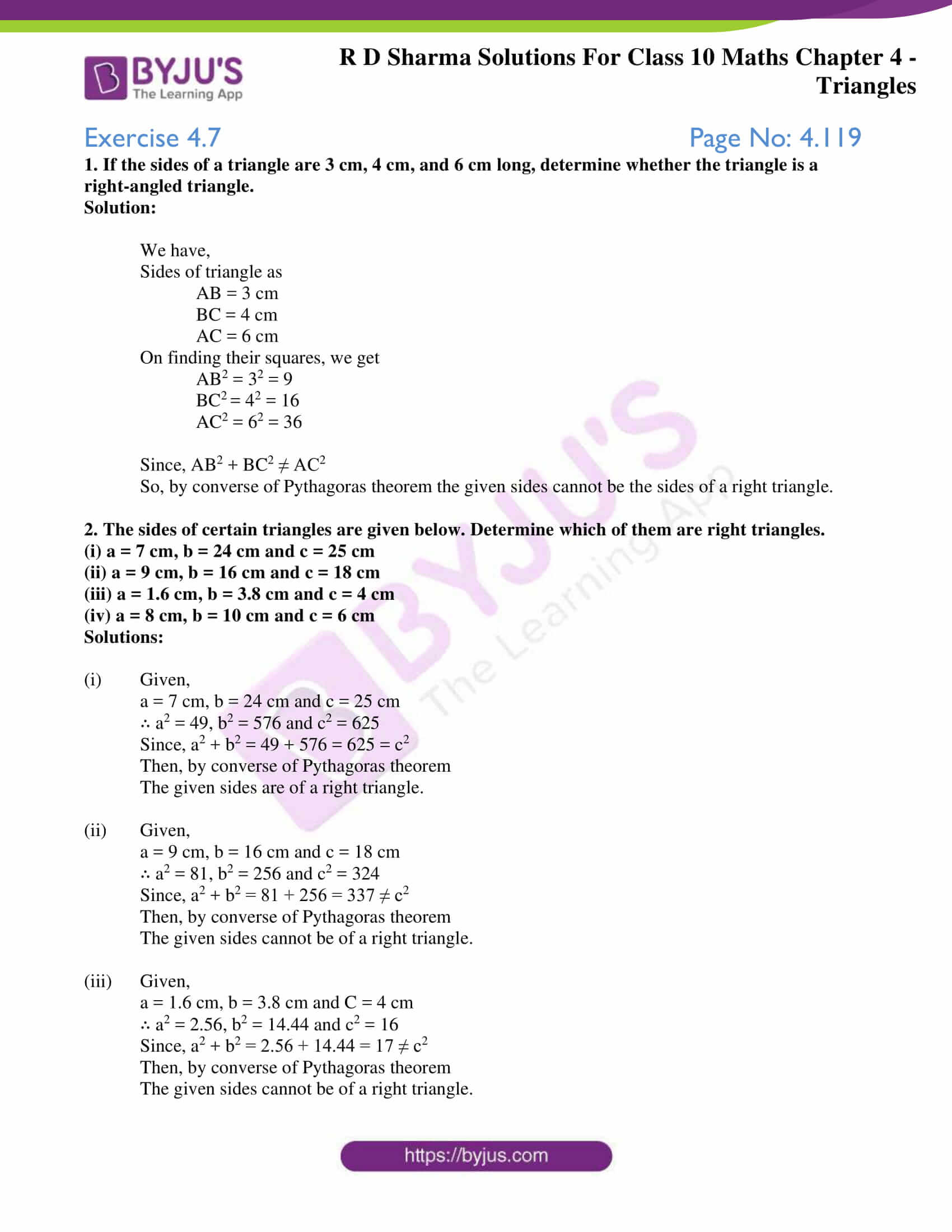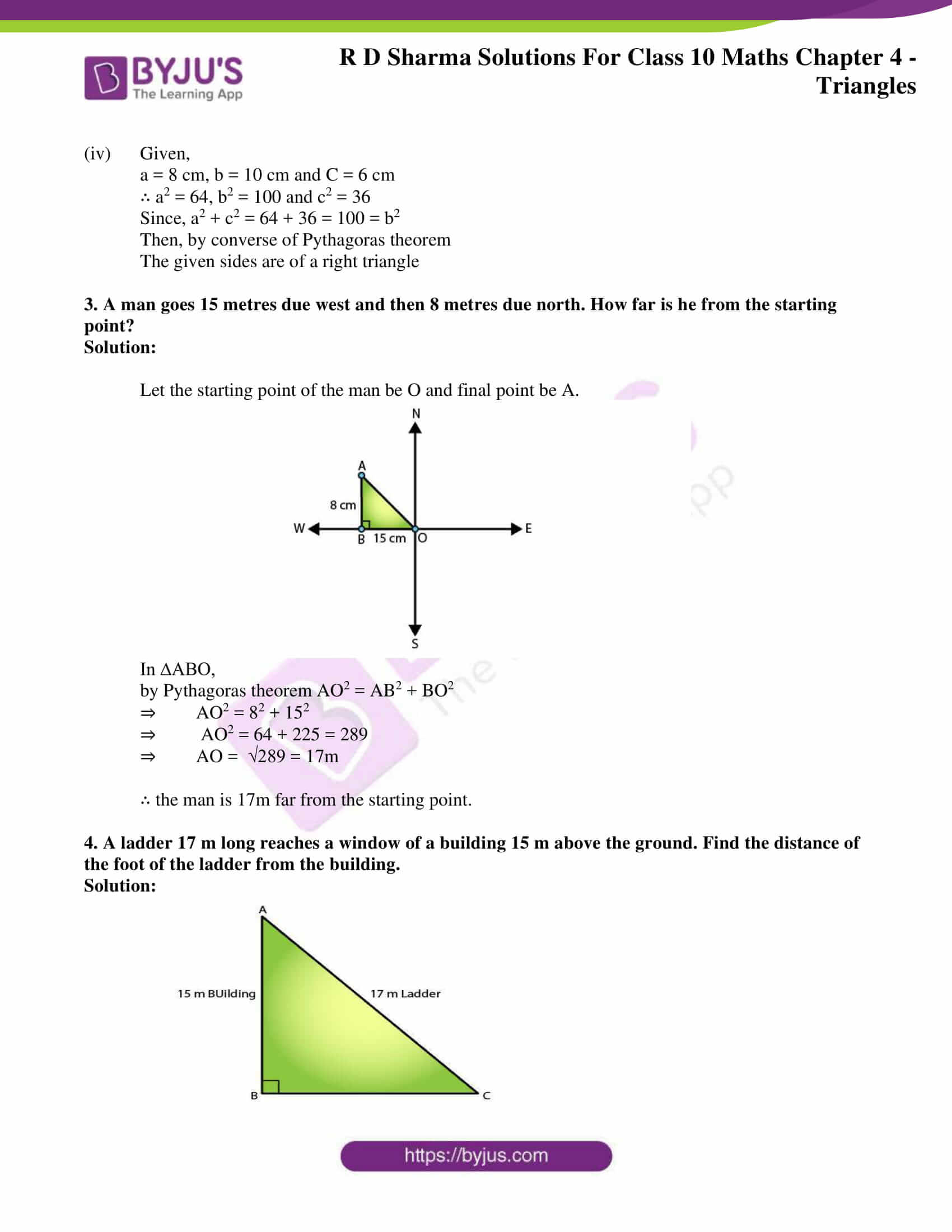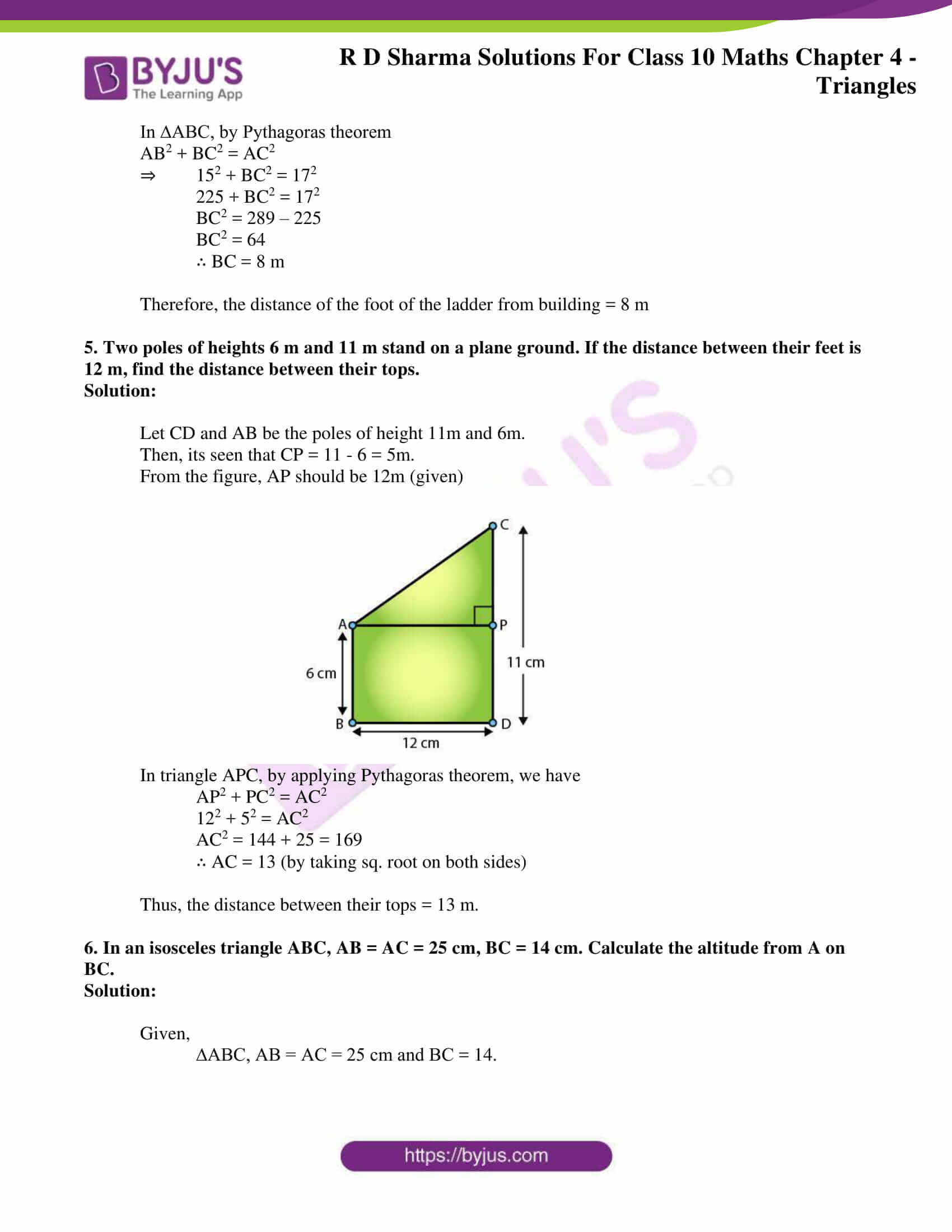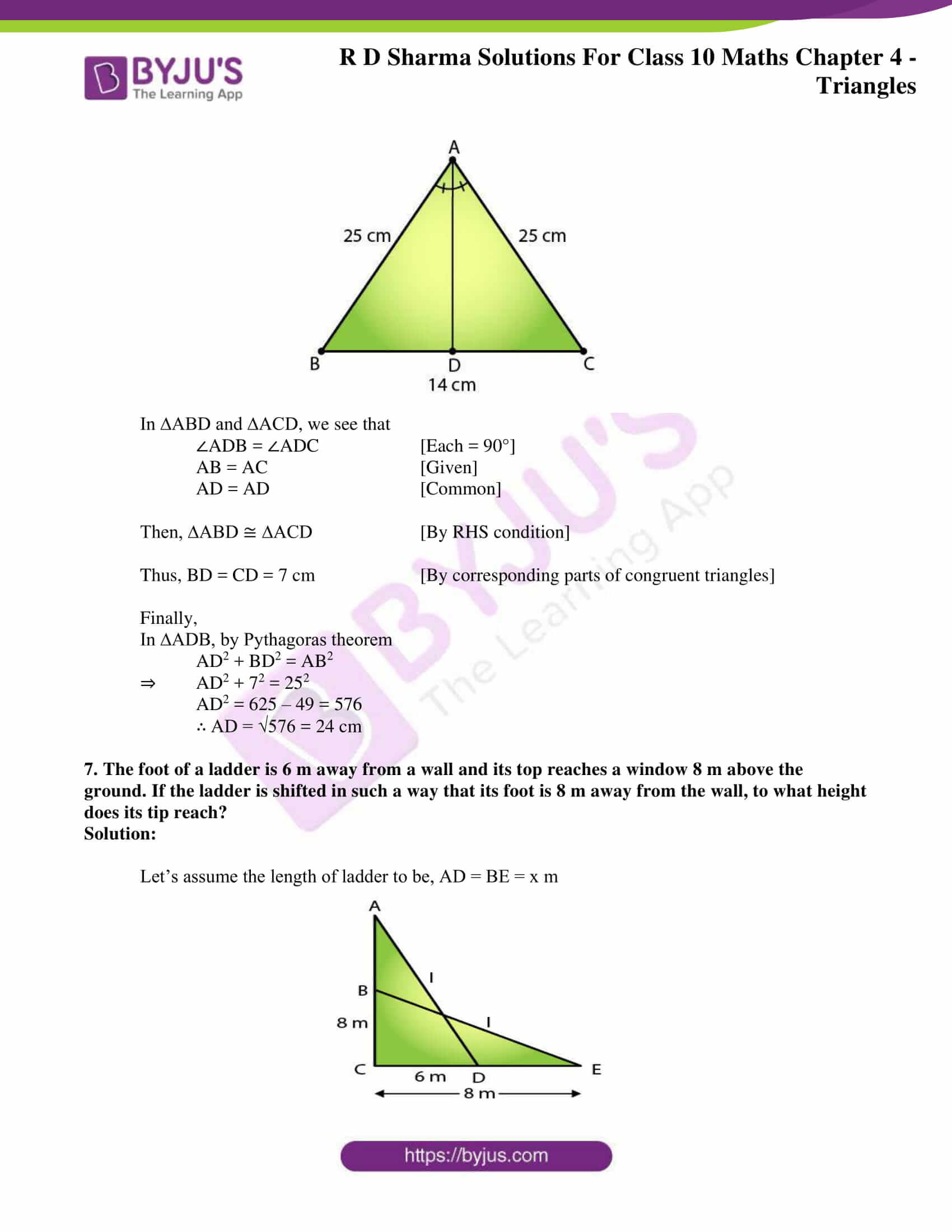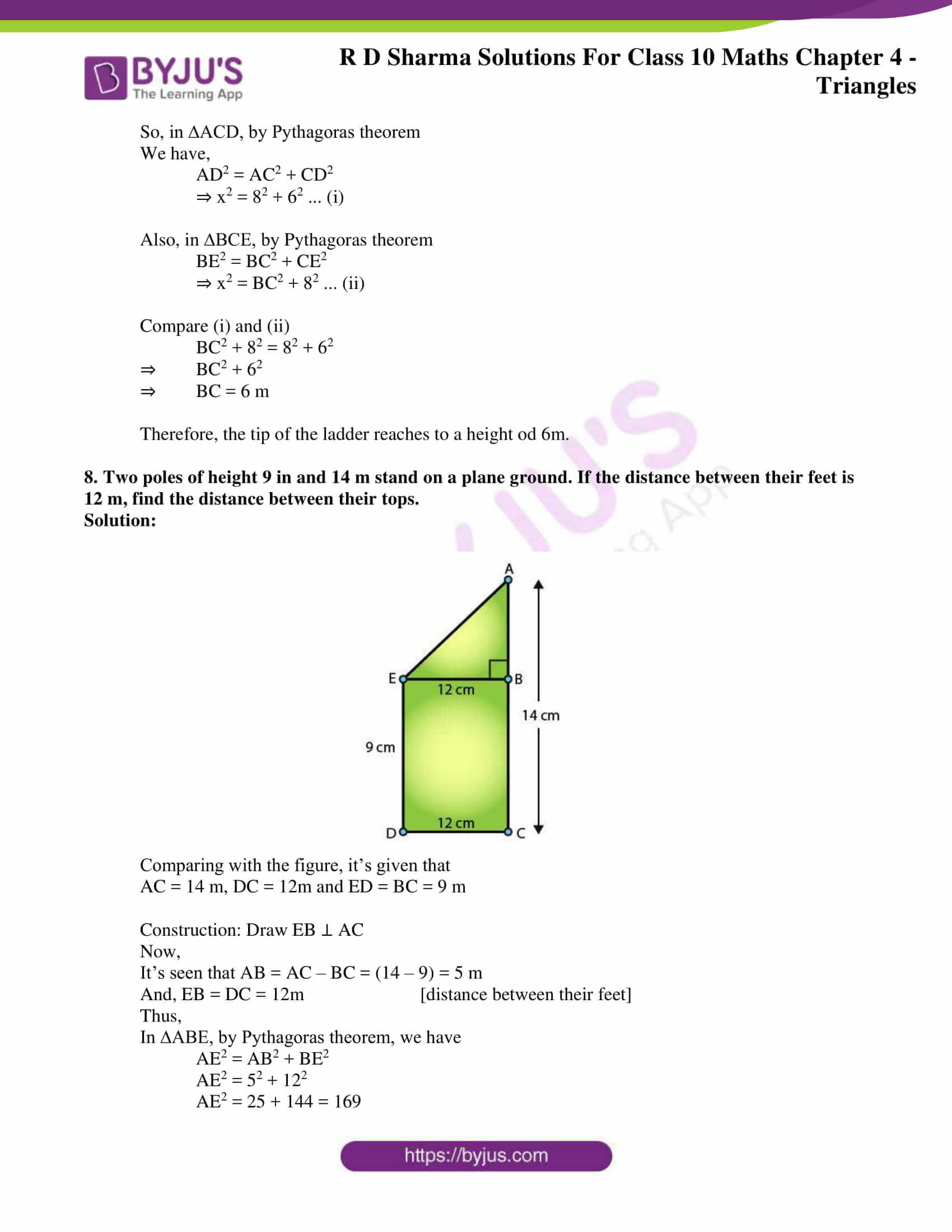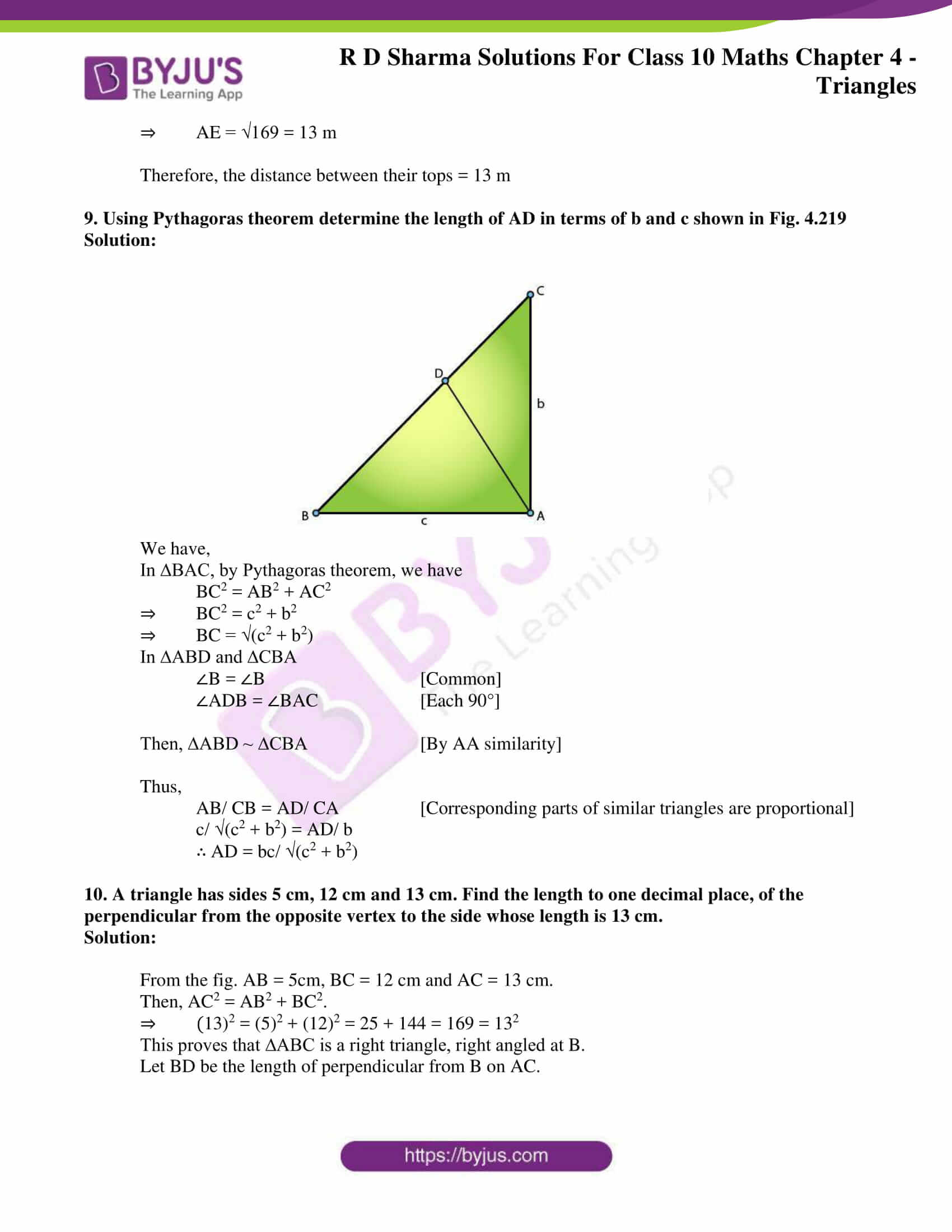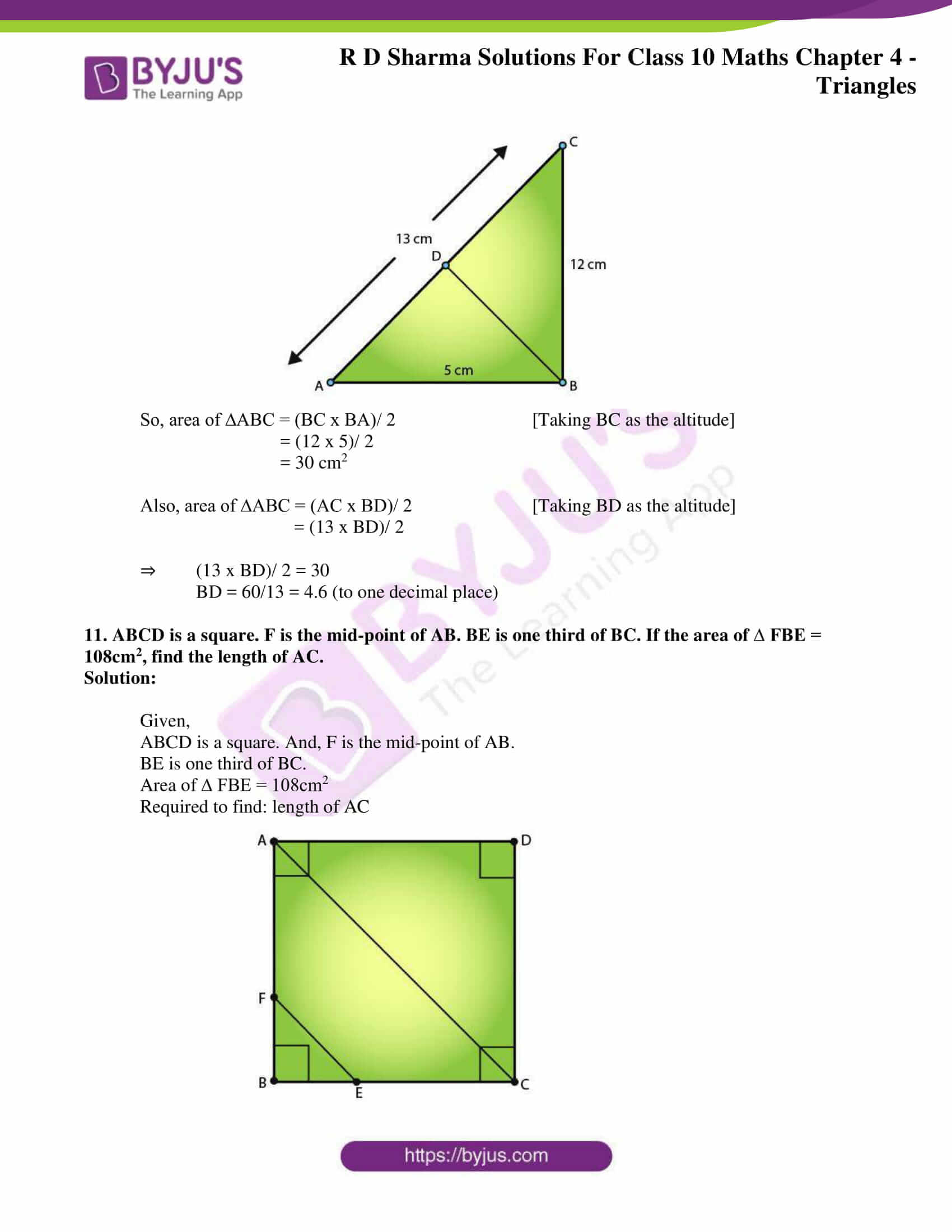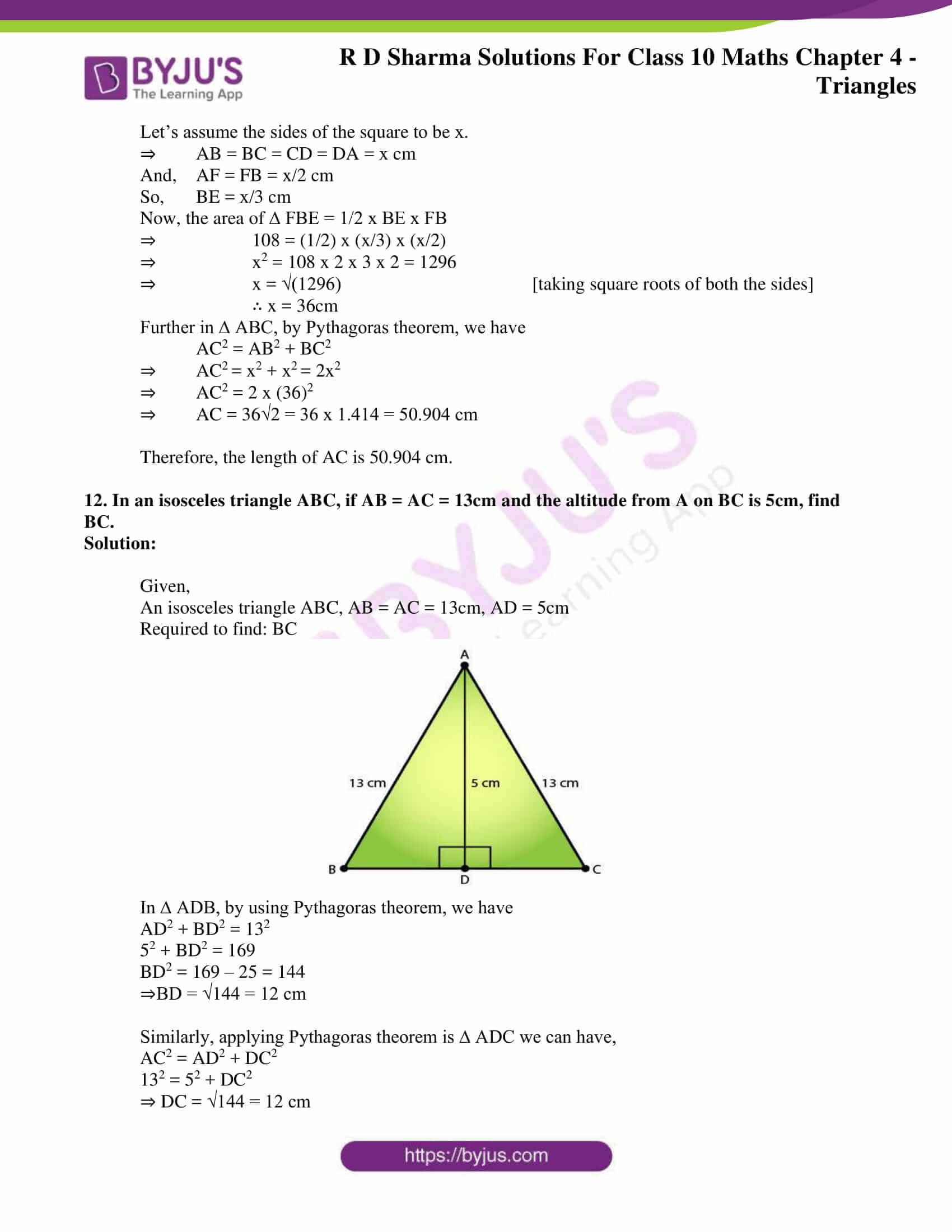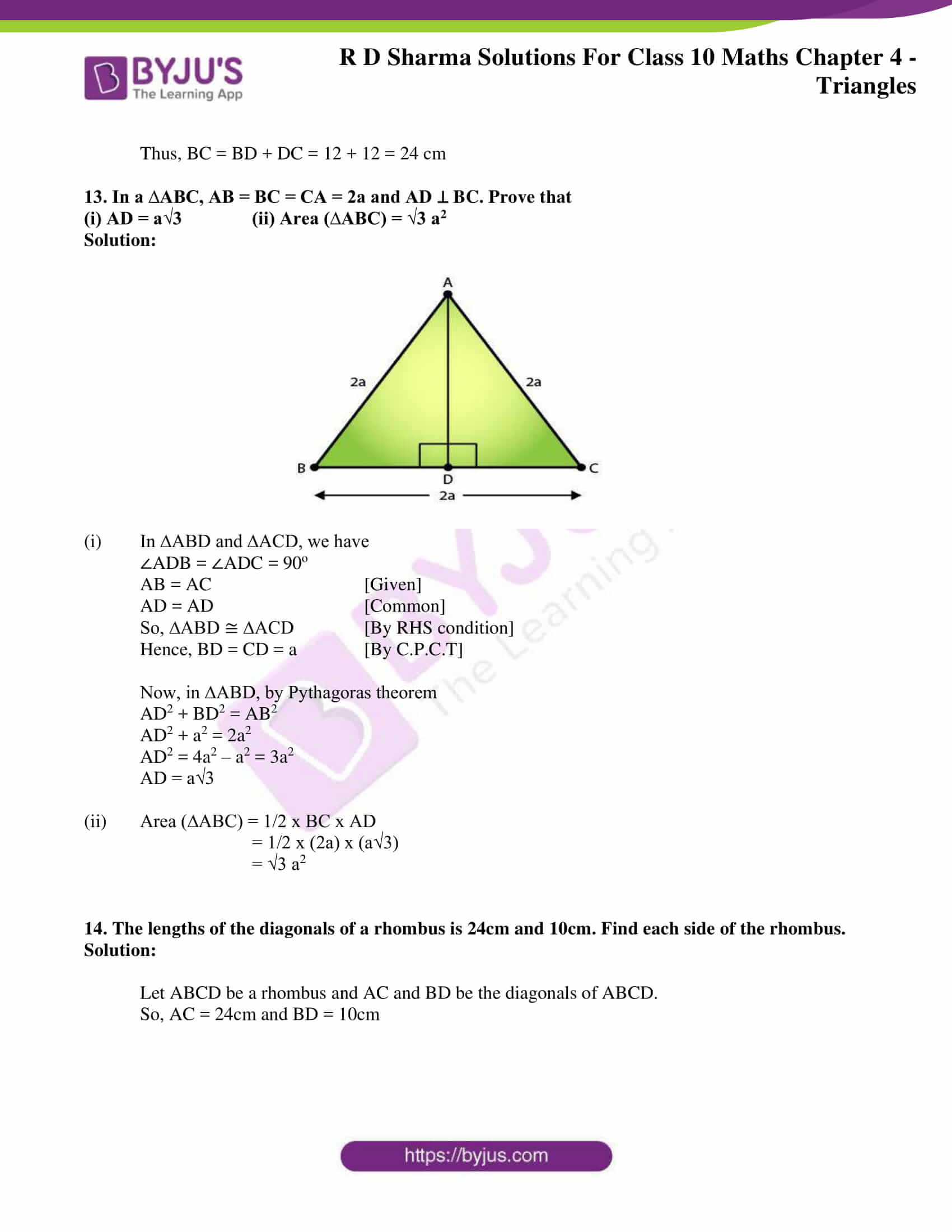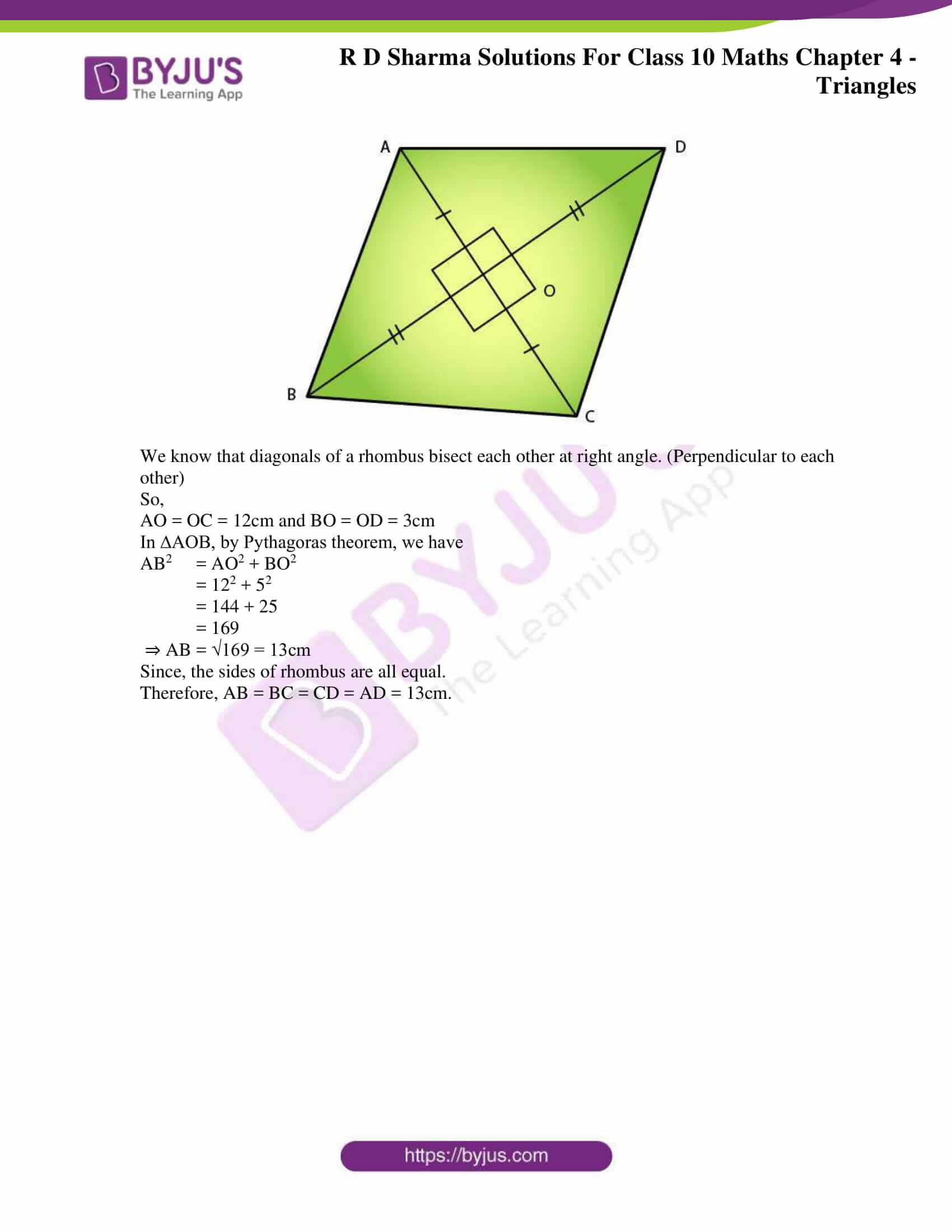### Access RD Sharma Solutions for Class 10 Maths Chapter 4 Triangles Exercise 4.7

1. If the sides of a triangle are 3 cm, 4 cm, and 6 cm long, determine whether the triangle is a right-angled triangle.

Solution:

We have,

Sides of triangle as

AB = 3 cm

BC = 4 cm

AC = 6 cm

On finding their squares, we get

AB2 = 32 = 9

BC= 42 = 16

AC2 = 62 = 36

Since, AB2 + BC2 ≠ AC2

So, by converse of Pythagoras theorem the given sides cannot be the sides of a right triangle.

2. The sides of certain triangles are given below. Determine which of them are right triangles.

(i) a = 7 cm, b = 24 cm and c = 25 cm

(ii) a = 9 cm, b = 16 cm and c = 18 cm

(iii) a = 1.6 cm, b = 3.8 cm and c = 4 cm

(iv) a = 8 cm, b = 10 cm and c = 6 cm

Solutions:

(i) Given,

a = 7 cm, b = 24 cm and c = 25 cm

∴ a2 = 49, b2 = 576 and c2 = 625

Since, a2 + b2 = 49 + 576 = 625 = c2

Then, by converse of Pythagoras theorem

The given sides are of a right triangle.

(ii) Given,

a = 9 cm, b = 16 cm and c = 18 cm

∴ a2 = 81, b2 = 256 and c2 = 324

Since, a2 + b2 = 81 + 256 = 337 ≠ c2

Then, by converse of Pythagoras theorem

The given sides cannot be of a right triangle.

(iii) Given,

a = 1.6 cm, b = 3.8 cm and C = 4 cm

∴ a2 = 2.56, b2 = 14.44 and c2 = 16

Since, a2 + b2 = 2.56 + 14.44 = 17 ≠ c2

Then, by converse of Pythagoras theorem

The given sides cannot be of a right triangle.

(iv) Given,

a = 8 cm, b = 10 cm and C = 6 cm

∴ a2 = 64, b2 = 100 and c2 = 36

Since, a2 + c2 = 64 + 36 = 100 = b2

Then, by converse of Pythagoras theorem

The given sides are of a right triangle

3. A man goes 15 metres due west and then 8 metres due north. How far is he from the starting point?

Solution:

Let the starting point of the man be O and final point be A.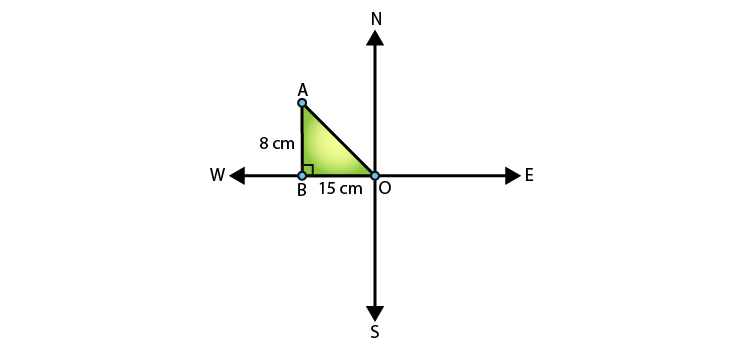In ∆ABO,

by Pythagoras theorem AO2 = AB2 + BO2

⇒ AO2 = 82 + 152

⇒ AO2 = 64 + 225 = 289

⇒ AO =  √289 = 17m

∴ the man is 17m far from the starting point.

4. A ladder 17 m long reaches a window of a building 15 m above the ground. Find the distance of the foot of the ladder from the building.

Solution: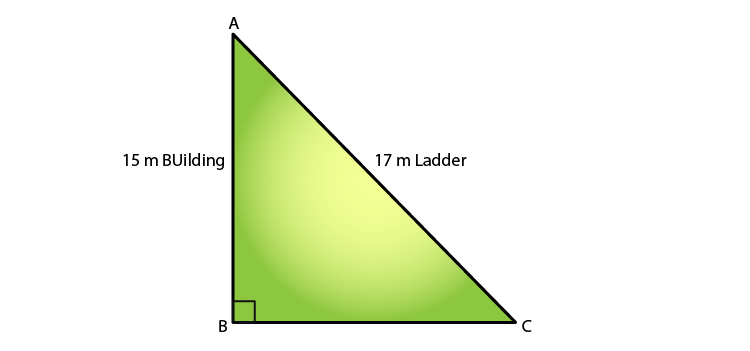In ∆ABC, by Pythagoras theorem

AB2 + BC2 = AC2

⇒ 152 + BC2 = 172

225 + BC2 = 172

BC2 = 289 – 225

BC2 = 64

∴ BC = 8 m

Therefore, the distance of the foot of the ladder from building = 8 m

5. Two poles of heights 6 m and 11 m stand on a plane ground. If the distance between their feet is 12 m, find the distance between their tops.

Solution:

Let CD and AB be the poles of height 11m and 6m.

Then, its seen that CP = 11 – 6 = 5m.

From the figure, AP should be 12m (given)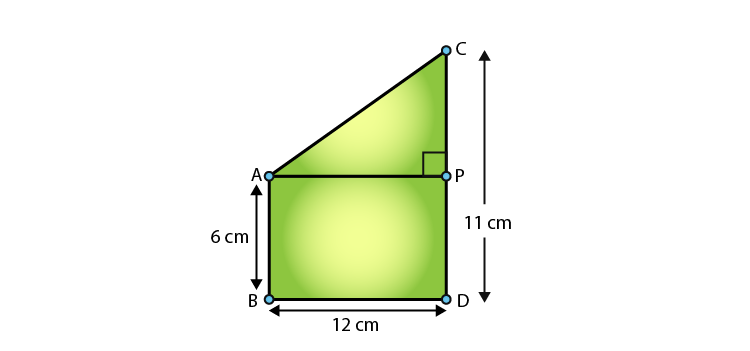In triangle APC, by applying Pythagoras theorem, we have

AP2 + PC2 = AC2

122 + 52 = AC2

AC2 = 144 + 25 = 169

∴ AC = 13 (by taking sq. root on both sides)

Thus, the distance between their tops = 13 m.

6. In an isosceles triangle ABC, AB = AC = 25 cm, BC = 14 cm. Calculate the altitude from A on BC.

Solution:

Given,

∆ABC, AB = AC = 25 cm and BC = 14.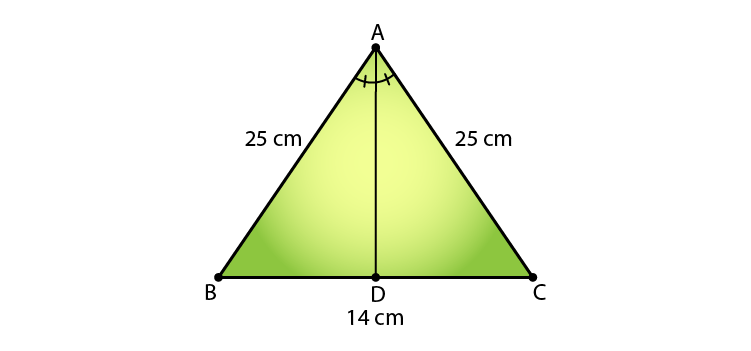In ∆ABD and ∆ACD, we see that

AB = AC                                [Given]

Then, ∆ABD ≅ ∆ACD      [By RHS condition]

Thus, BD = CD = 7 cm              [By corresponding parts of congruent triangles]

Finally,

⇒ AD2 + 72 = 252

AD2 = 625 – 49 = 576

∴ AD = √576 = 24 cm

7. The foot of a ladder is 6 m away from a wall and its top reaches a window 8 m above the ground. If the ladder is shifted in such a way that its foot is 8 m away from the wall, to what height does its tip reach?

Solution:

Let’s assume the length of ladder to be, AD = BE = x m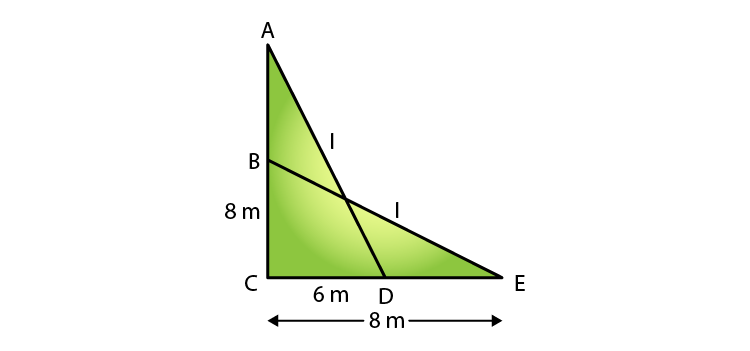So, in ∆ACD, by Pythagoras theorem

We have,

⇒ x2 = 82 + 62 … (i)

Also, in ∆BCE, by Pythagoras theorem

BE2 = BC2 + CE2

⇒ x2 = BC2 + 82 … (ii)

Compare (i) and (ii)

BC2 + 82 = 82 + 62

⇒ BC2 + 62

⇒ BC = 6 m

Therefore, the tip of the ladder reaches to a height od 6m.

8. Two poles of height 9 in and 14 m stand on a plane ground. If the distance between their feet is 12 m, find the distance between their tops.

Solution: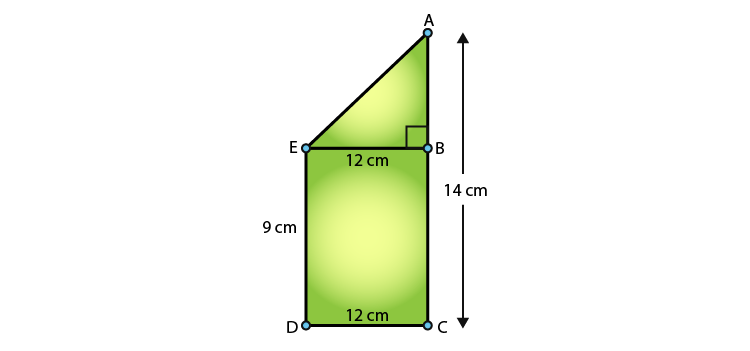Comparing with the figure, it’s given that

AC = 14 m, DC = 12m and ED = BC = 9 m

Construction: Draw EB ⊥ AC

Now,

It’s seen that AB = AC – BC = (14 – 9) = 5 m

And, EB = DC = 12m [distance between their feet]

Thus,

In ∆ABE, by Pythagoras theorem, we have

AE2 = AB2 + BE2

AE2 = 52 + 122

AE2 = 25 + 144 = 169

⇒ AE = √169 = 13 m

Therefore, the distance between their tops = 13 m

9. Using Pythagoras theorem determine the length of AD in terms of b and c shown in Fig. 4.219

Solution: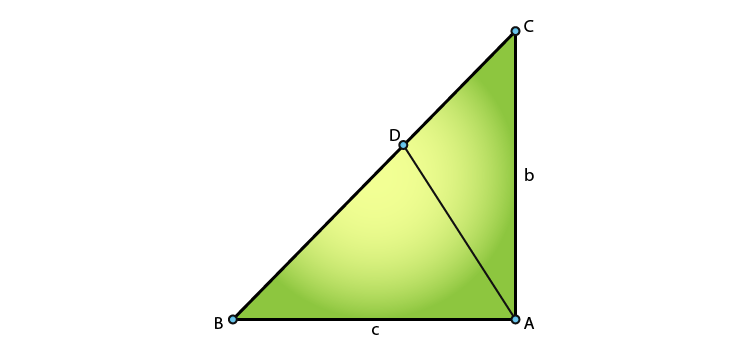We have,

In ∆BAC, by Pythagoras theorem, we have

BC2 = AB2 + AC2

⇒ BC2 = c2 + b2

⇒ BC = √(c2 + b2)

In ∆ABD and ∆CBA

∠B = ∠B                      [Common]

Then, ∆ABD ͏~ ∆CBA   [By AA similarity]

Thus,

AB/ CB = AD/ CA [Corresponding parts of similar triangles are proportional]

c/ √(c2 + b2) = AD/ b

∴ AD = bc/ √(c2 + b2)

10. A triangle has sides 5 cm, 12 cm and 13 cm. Find the length to one decimal place, of the perpendicular from the opposite vertex to the side whose length is 13 cm.

Solution:

From the fig. AB = 5cm, BC = 12 cm and AC = 13 cm.

Then, AC2 = AB2 + BC2.

⇒ (13)2 = (5)2 + (12)2 = 25 + 144 = 169 = 132

This proves that ∆ABC is a right triangle, right angled at B.

Let BD be the length of perpendicular from B on AC.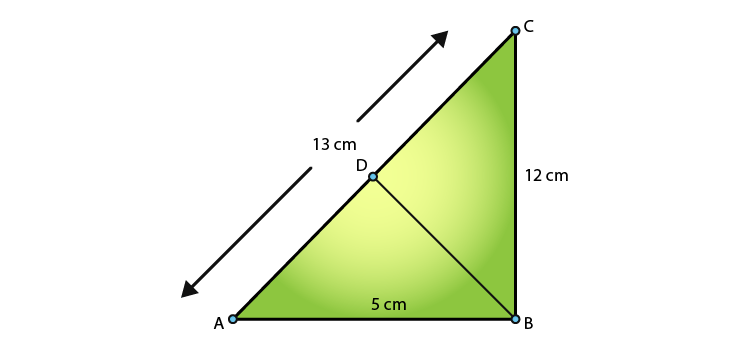So, area of ∆ABC = (BC x BA)/ 2 [Taking BC as the altitude]

= (12 x 5)/ 2

= 30 cm2

Also, area of ∆ABC = (AC x BD)/ 2 [Taking BD as the altitude]

= (13 x BD)/ 2

⇒ (13 x BD)/ 2 = 30

BD = 60/13 = 4.6 (to one decimal place)

11. ABCD is a square. F is the mid-point of AB. BE is one third of BC. If the area of ∆ FBE = 108cm2, find the length of AC.

Solution:

Given,

ABCD is a square. And, F is the mid-point of AB.

BE is one third of BC.

Area of ∆ FBE = 108cm2

Required to find: length of AC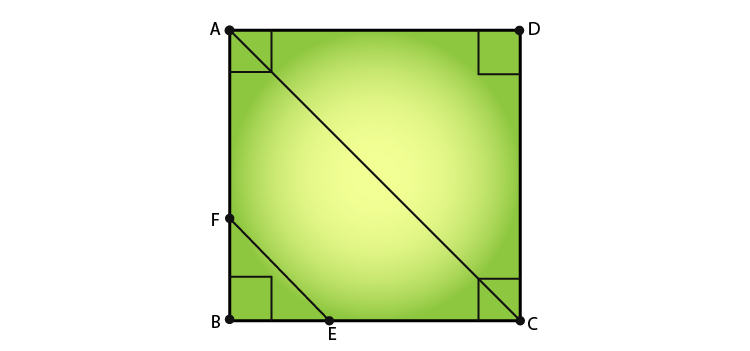Let’s assume the sides of the square to be x.

⇒ AB = BC = CD = DA = x cm

And, AF = FB = x/2 cm

So, BE = x/3 cm

Now, the area of ∆ FBE = 1/2 x BE x FB

⇒ 108 = (1/2) x (x/3) x (x/2)

⇒ x2 = 108 x 2 x 3 x 2 = 1296

⇒ x = √(1296) [taking square roots of both the sides]

∴ x = 36cm

Further in ∆ ABC, by Pythagoras theorem, we have

AC2 = AB2 + BC2

⇒ AC2 = x2 + x2 = 2x2

⇒ AC2 = 2 x (36)2

⇒ AC = 36√2 = 36 x 1.414 = 50.904 cm

Therefore, the length of AC is 50.904 cm.

12. In an isosceles triangle ABC, if AB = AC = 13cm and the altitude from A on BC is 5cm, find BC.

Solution:

Given,

An isosceles triangle ABC, AB = AC = 13cm, AD = 5cm

Required to find: BC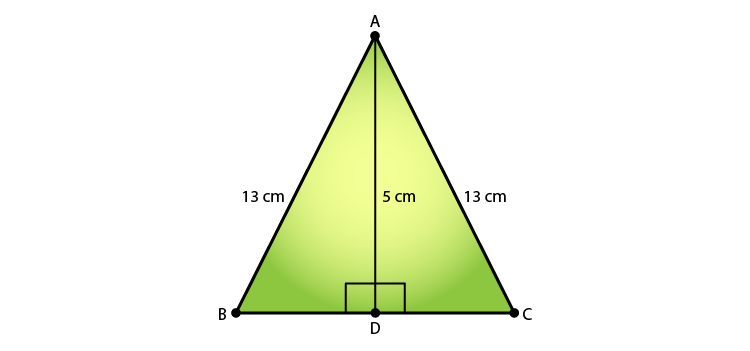In ∆ ADB, by using Pythagoras theorem, we have

52 + BD2 = 169

BD2 = 169 – 25 = 144

⇒BD = √144 = 12 cm

Similarly, applying Pythagoras theorem is ∆ ADC we can have,

132 = 52 + DC2

⇒ DC = √144 = 12 cm

Thus, BC = BD + DC = 12 + 12 = 24 cm

13. In a ∆ABC, AB = BC = CA = 2a and AD ⊥ BC. Prove that

(i) AD = a√3 (ii) Area (∆ABC) = √3 a2

Solution: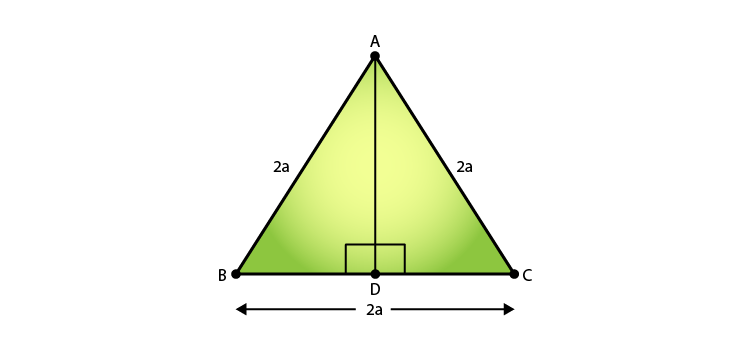(i) In ∆ABD and ∆ACD, we have

AB = AC     [Given]

So, ∆ABD ≅ ∆ACD   [By RHS condition]

Hence, BD = CD = a [By C.P.C.T]

Now, in ∆ABD, by Pythagoras theorem

AD2 = 4a2 – a2 = 3a2

(ii) Area (∆ABC) = 1/2 x BC x AD

= 1/2 x (2a) x (a√3)

= √3 a2

14. The lengths of the diagonals of a rhombus is 24cm and 10cm. Find each side of the rhombus.

Solution:

Let ABCD be a rhombus and AC and BD be the diagonals of ABCD.

So, AC = 24cm and BD = 10cm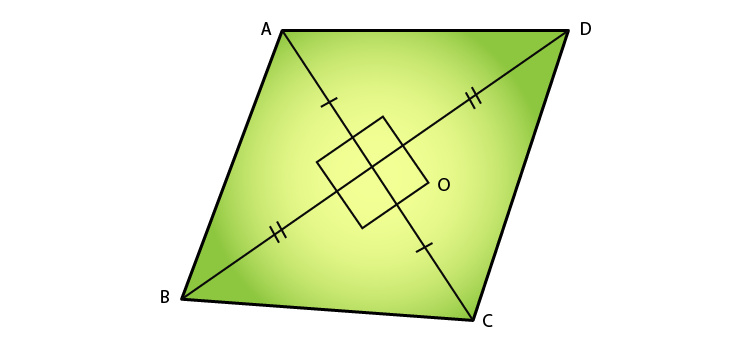We know that diagonals of a rhombus bisect each other at right angle. (Perpendicular to each other)

So,

AO = OC = 12cm and BO = OD = 3cm

In ∆AOB, by Pythagoras theorem, we have

AB2 = AO2 + BO2

= 122 + 52

= 144 + 25

= 169

⇒ AB = √169 = 13cm

Since, the sides of rhombus are all equal.

Therefore, AB = BC = CD = AD = 13cm.